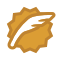# 简单计数器

当两个线程同时访问synchronized 同步代码块是只能有一个线程得到执行 另外一个进程必须等到当前代码执行完才可以执行

<%@ page language="java" contentType="text/html; charset=UTF-8"
pageEncoding="UTF-8"%>

<%
Integer count = null;   //Integer 是一个类 int是一个基本类型
synchronized(application)
{
//从内存中读取访问量
count = (Integer)application.getAttribute("basic.counter");
if(count == null)
count = new Integer(0);
count = new Integer(count.intValue() + 1); //开始计数
application.setAttribute("basic.counter", count);
}
%>
<html>
<title>简单计数器</title>
<body>
<center>
<font  size = 5 color = blue >简单计数器</font>
<br>
<hr>
<br>
<font size = 6 color = blue> 您是本站第<%= count %>位客人!</font>
</center>
</body>
</html>

## 多边形重心问题

3
3
0 1
0 2
0 3
3
1 1
0 0
0 1
4
1 1
0 0
0 0.5
0 1

0.000 0.000
0.500 1.000
0.500 1.000

#include<iostream>
#include<cstdio>
#include<cmath>
#include<algorithm>
using namespace std;
const int  N = 100010;
struct Point
{
double x, y;
Point():x(0), y(0){}
}p[N];

int main()
{
int ncase , m ;
scanf("%d", &ncase);
while(ncase--)
{
scanf("%d", &m);
for(int i = 0 ; i < m ; i++)
{
scanf("%lf%lf", &p[i].x, &p[i].y);
}
//上面是输入部分，选择要想的是如何可以计算重心
//根据将多边形分解成三角形 计算三角形面积
//如何把面积和重心联系在一起，自己百度吧， 文库里面有个专门论文写的就是这个
double result = 0;   //用来记录面积
Point ans;       //用来记录最终重心的位置与面积的乘积 而且已经初始化为(0,0)了
for(int i = 1 ; i <= m ; i++ )
{//为什么要%m 是为了要组成一个封闭的图案最后那个是m %m 就是0 最后就是结果封闭了
double  temp =(p[i%m].x * p[i].y - p[i-1].x*p[i%m].y)/2.0;    //首先记录三角形面积x2*y1 - x1*y2 即可进行求解
result += temp;
ans.x  += temp*(p[i%m].x + p[i-1].x)/3.0;
ans.y  += temp*(p[i%m].y + p[i-1].y)/3.0;
}
//下面输出面积即可
printf("%.3lf %.3lf\n", fabs(result), (ans.x + ans.y)/result);

}
return 0;
}


wangxiaomingCSDN认证博客专家 架构 Spring Boot Redis

11-02507

#### 例2-4通过synchronized方法操作一个成员变量来实现一个简单的计数器06-13349

#### synchronized简单示例

08-121355

#### java中Synchronized的一个简单例子

04-01472

#### JSP——通过一个synchronized方法操作一个成员变量来实现一个简单的计数器。

10-22521

#### 【转】简单计数器以及synchronized 关键字

01-13179

#### vue入门 实现简易计数器©️2020 CSDN 皮肤主题: Age of Ai 设计师: meimeiellie点击重新获取扫码支付1.余额是钱包充值的虚拟货币，按照1:1的比例进行支付金额的抵扣。
2.余额无法直接购买下载，可以购买VIP、C币套餐、付费专栏及课程。余额充值